The nested Platonic Solids can be elegantly represented in the Rhombic Triacontahedron, as shown in Rhombic Triacontahedron.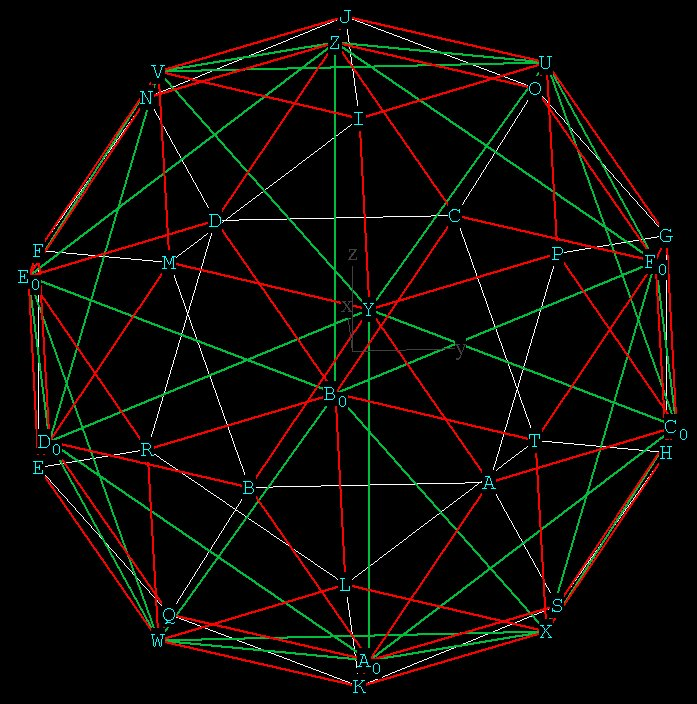Figure 1 -- the Rhombic Triacontahedron in red with its  Phi Ratio rhombi, the Icosahedron in green with its equilateral triangle faces, and the Dodecahedron in white with its pentagonal faces. The Rhombic Triacontahedron is itself a combination of the Icosahedron and the Dodecahedron, and it demonstrates the proper relationship between the 5 nested Platonic Solids.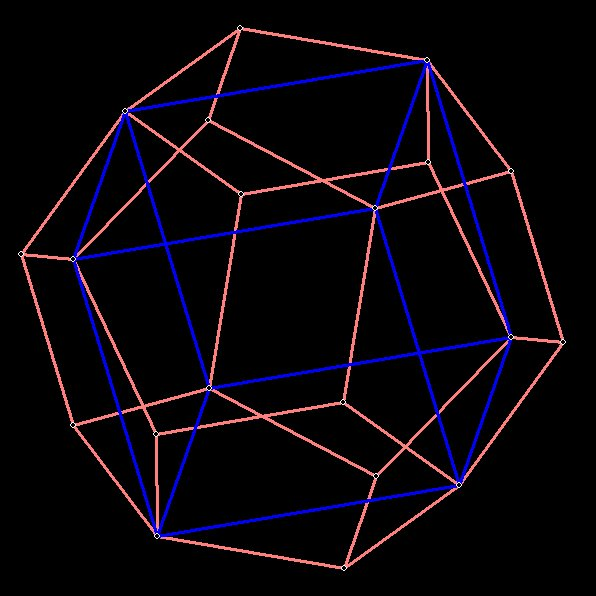Figure 2 --
The cube fits quite nicely within the dodecahedron, as shown above. The cube has 8 vertices and 5 different cubes will fit within the dodecahedron. Each cube has 12 edges, and each edge will be a diagonal of one of the 12 pentagonal faces of the dodecahedron. Since there are only 5 diagonals to a pentagon, there can only be 5 different cubes, each of which will be angled 36 degrees from each other.
(Why is this? Because the diagonals of the pentagon are angled 36 degrees from each other)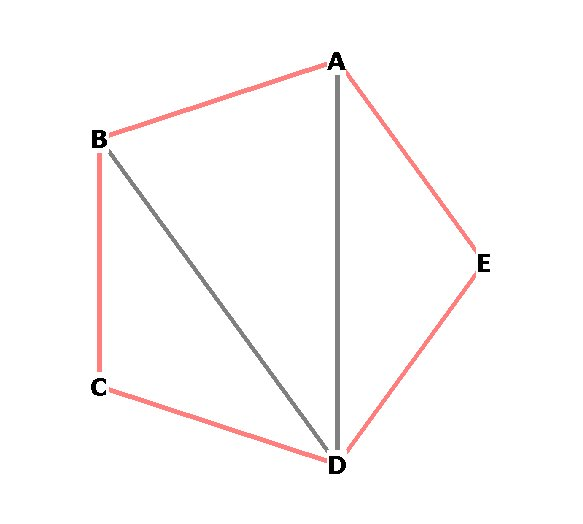Figure 3 --
Angle BDA is 36 degrees. Triangle BDA is a 36-72-72 golden triangle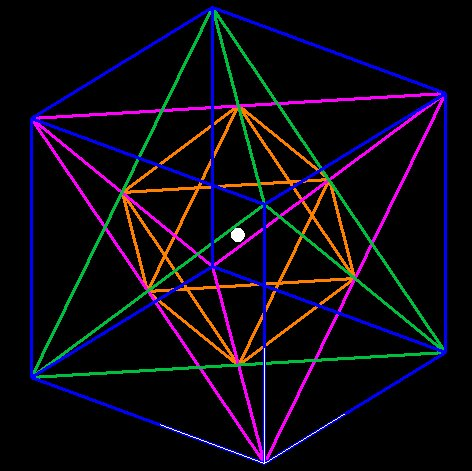Figure 4 --
The tetrahedron and the octahedron fit nicely within the cube, as shown above

So the Platonic Solid nesting order as given by the Rhombic Triacontahedron goes as follows: Icosahedron, Dodecahedron, Cube, Tetrahedron, Octahedron. Here's how the whole thing looks, all enclosed within a sphere: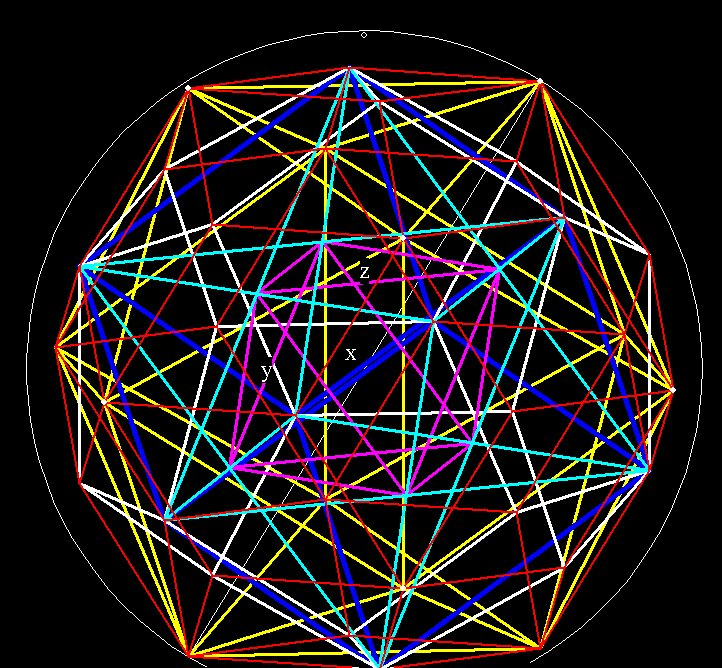Figure 5 --
The 5 nested Platonic Solids inside a sphere. The Icosahedron in cream, the rhombic triacontahedron in red, the dodecahedron in white, the cube in blue, 2 interlocking tetrahedra in cyan, and the octahedron in magenta. Only the 12 vertices of the icosahedron touch the sphere boundary.Figure 5A -- Figure 5 as an animated GIF. This is 2.7 Meg file, but I've included it for those of you with fast download speeds. It's much easier to distinguish the 5 polyhedra as an animation.

Surprisingly, even though there are 5 Platonic solids, there are only 3 different spheres which contain them. That is because the 4 vertices of each tetrahedron are 4 of the 8 cube vertices, and the 8 vertices of the cube are 8 of the 12 vertices of the dodecahedron.

Figure 4 shows that the octahedron is formed from the intersecting lines of the 2 interlocking tetrahedrons. The edges of the tetrahedrons are just the diagonals of the cube faces, and the intersection of the two tetrahedron edges meet precisely at the midpoint of the cube face (the centroid). If you look at Figure 3 you'll see how the green and purple lines intersect precisely in the middle of the cube face, making an "X."

If we let the radius of the sphere which encloses the octahedron = 1, then what is the radius of the other two spheres?
Since the octahedron is formed from the midpoints of all of the cube faces, the sphere which encloses it fits precisely within the cube, like so:Figure 6 --
The radius of the circle which encloses the octahedron we will arbitrarily set = 1.

One sphere encloses both the cube and the dodecahedron: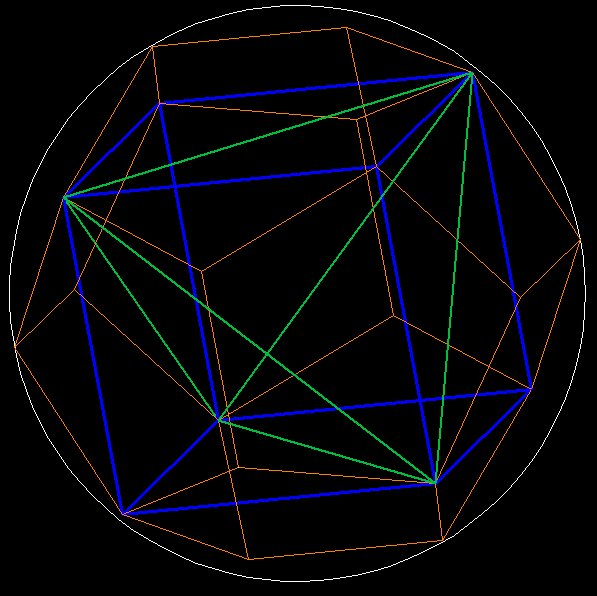Figure 7 --
The sphere which encloses both dodecahedron and cube.
The radius of this sphere is \/¯ ­3 times the sphere which encloses the octahedron.
The two spheres are the in-sphere of the cube and the circumsphere of the cube.
The outer sphere which encloses the icosahedron is slightly larger; \/¯(Ø² + 1) / \/¯3 larger, in fact!

So the radii of the three enclosing spheres is: 1, \/¯3,  \/¯(Ø² + 1).

Special characters:
\/¯ ­ ° ¹ ² ³ × ½ ¼ Ø  \/¯(Ø² + 1)